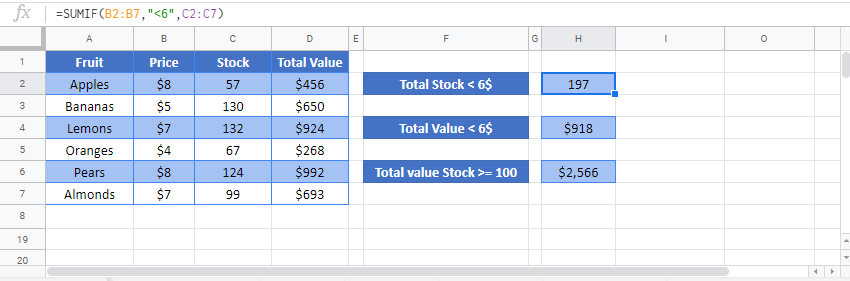# SUMIF & SUMIFS Functions – Sum Values If – Excel & Google Sheets

This tutorial demonstrates how to use the Excel SUMIF and SUMIFS Functions in Excel and Google Sheets to sum data that meet certain criteria.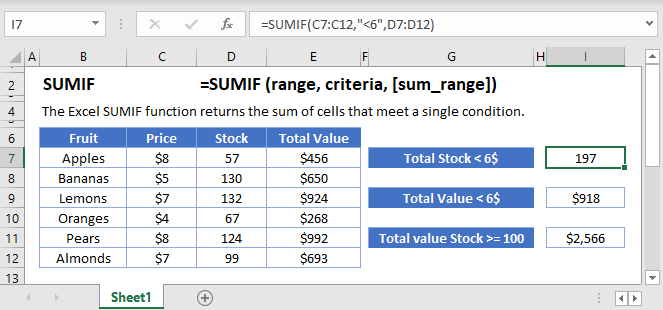## SUMIF Function Overview

You can use the SUMIF function in Excel to sum of cells that contain a specific value, sum cells that are greater than or equal to a value, etc.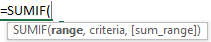(Notice how the formula inputs appear)

## SUMIF Function Syntax and Arguments:

``=SUMIF (range, criteria, [sum_range])``

range – The range of cells that you want to apply the criteria against.

criteria – The criteria used to determine which cells to add.

sum_range – [optional] The cells to add together. If sum_range is omitted, the cells in range are added together instead.

## What is the SUMIF function?

The SUMIF function is one of the older functions used in spreadsheets. It is used to scan through a range of cells checking for a specific criterion, and then adding up values in a range that correspond to those values. The original SUMIF function was limited to just one criterion. After 2007, the SUMIFS function was created which allows a multitude of criteria. Most of the general use remains the same between the two, but there are some critical differences in the syntax that we’ll discuss throughout this article.

If you haven’t already, you can review much of the similar structure and examples in the COUNTIFS article <insert link>.

### Basic example

Let’s consider this list of recorded sales, and we want to know the total income.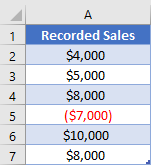Because we had an expense, the negative value, we can’t just do a basic sum. Instead, we want to sum only the values that are greater than 0. The “greater than 0” is what will be our criteria in a SUMIF function. Our formula to state this is

``=SUMIF(A2:A7, ">0")``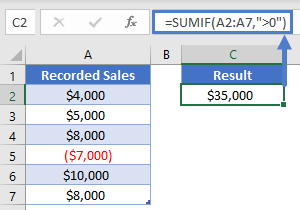### Two-column example

While the original SUMIF function was designed to let you apply a criterion to the range of numbers you want to sum, much of the time you’ll need to apply one or more criteria to other columns. Let’s consider this table: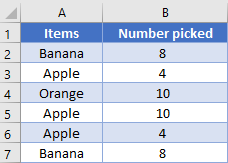Now, if we use the original SUMIF function to find out how many Bananas we have (listed in cell D1), we’ll need to give the range we want to sum as the last argument, and so our formula would be

``=SUMIF(A2:A7, D1, B2:B7)``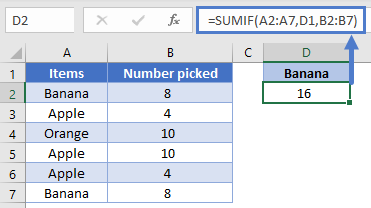However, when programmers eventually realized that users wanted to give more than one criterion, the SUMIFS function was created. In order to create one structure that would work for any number of criteria, the SUMIFS requires that the sum range is listed first. In our example, this means that the formula needs to be

``=SUMIFS(B2:B7, A2:A7, D1)``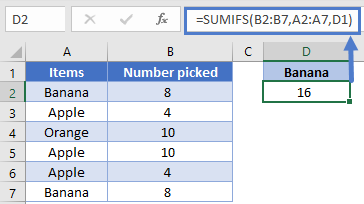NOTE: These two formulas get the same result and can look similar, so pay close attention to which function is being used to make sure you list out all the arguments in the correct order.

### Working with Dates, Multiple criteria

When working with dates in a spreadsheet, while it is possible to input the date directly into the formula, it’s best practice to have the date in a cell so that you can just reference the cell in a formula. For example, this helps the computer know that you’re wanting to use the date 5/27/2020, and not the number 5 divided by 27 divided by 2020.

Let’s look at our next table recording the number of visitors to a site every two weeks.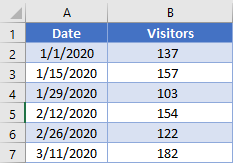We can specify the start and end points of the range we want to look at in D2 and E2. Our formula then to sum the number of visitors in this range could be:

``=SUMIFS(B2:B7, A2:A7, ">="&D2, A2:A7, "<="&E2)``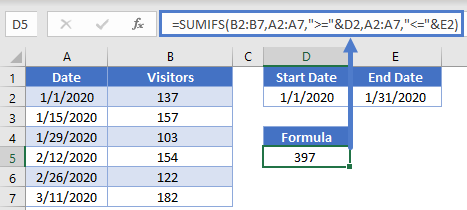Note how we were able to concatenate the comparisons of “<=” and “>=” to the cell references to create the criteria. Also, even though both criteria were being applied to the same range of cells (A2:A7), you need to write out the range twice, once per each criterion.

### Easy Excel Automation### Multiple columns

When using multiple criteria, you can apply them to the same range as we did with previous example, or you can apply them to different ranges. Let’s combine our sample data into this table: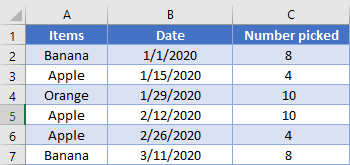We’ve setup some cells for the user to enter what they want to search for in cells E2 through G2. We thus need a formula that will add up the total number of apples picked in February. Our formula looks like this:

``=SUMIFS(C2:C7, B2:B7, ">="&F2, B2:B7, "<="&G2, A2:A7, E2)``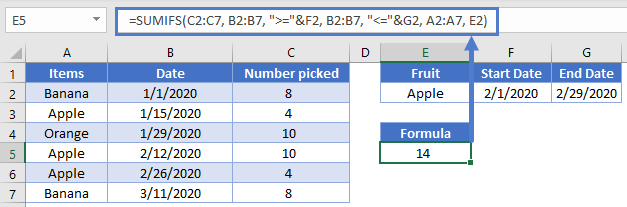### SUMIFS with OR type logic

Up to this point, the examples we’ve used have all been AND based comparison, where we are looking for rows that meet all our criteria. Now, we’ll consider the case when you want to search for the possibility of a row meeting one or another criterion.

Let’s look at this list of sales: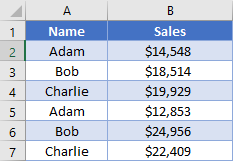We’d like to add up the total sales for both Adam and Bob. To do this, you have a couple of options. The simplest is to add two SUMIFS together, like so:

``=SUMIFS(B2:B7, A2:A7, "Adam")+SUMIFS(B2:B7, A2:A7, "Bob")``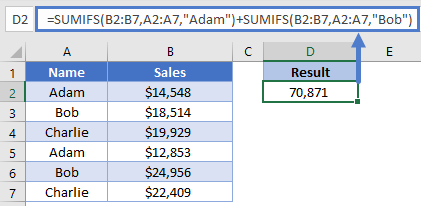Here, we’ve had the computer calculate our individual scores, and then we add them together.

Our next option is good for when you have more criteria ranges, such that you don’t want to have to rewrite the whole formula repeatedly. In the previous formula, we manually told the computer to add two different SUMIFS together. However, you can also do this by writing your criteria inside an array, like this:

``=SUM(SUMIFS(B2:B7, A2:A7, {"Adam", "Bob"}))``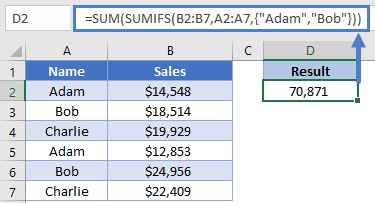Look at how the array is constructed inside the curly brackets. When the computer evaluates this formula, it will know that we want to calculate a SUMIFS function for each item in our array, thus creating an array of numbers. The outer SUM function will then take that array of numbers and turn it into a single number. Stepping through the formula evaluation, it would look like this:

``````=SUM(SUMIFS(B2:B7, A2:A7, {"Adam", "Bob"}))
=SUM(27401, 43470)
=70871``````

We get the same result, but we were able to write out the formula a bit more succinctly.

### Dealing with blanks

Sometimes your data set will have blank cells that you need to either find or avoid. Setting up the criteria for these can be a little tricky, so let’s look at another example.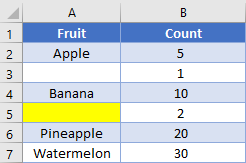Note that cell A3 is truly blank, while cell A5 has a formula returning a zero-length string of “”. If we want to find the total sum of truly blank cells, we’d use a criterion of “=”, and our formula would look like this:

``=SUMIFS(B2:B7,A2:A7,"=")``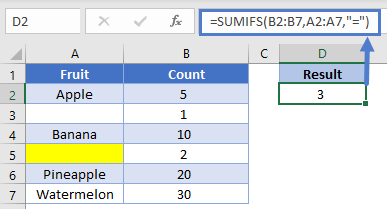On the other hand, if we want to get the sum for all cells that visually looks blank, we’ll change the criteria to be “”, and the formula looks like

``=SUMIFS(B2:B7,A2:A7,"")``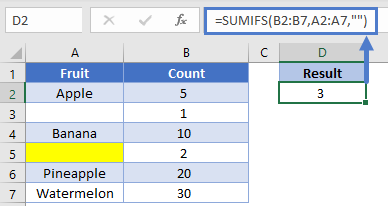Let’s flip it around: what if you want to find the sum of non-blank cells? Unfortunately, the current design won’t let you avoid the zero-length string. You can use a criterion of “<>”, but as you can see in the example, it still includes the value from row 5.

``=SUMIFS(B2:B7,A2:A7,"<>")``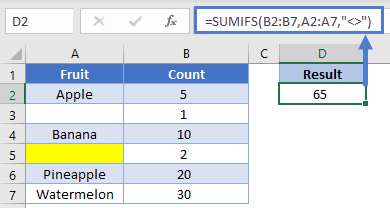If you need to not count cells containing zero length strings, you’ll want to consider using the LEN function inside a SUMPRODUCT <link to SUMPRODUCT article).